# Java Regular Expressions Tutorial with Examples

#### View more Tutorials: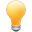Follow us on our fanpages to receive notifications every time there are new articles.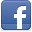Facebook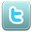Twitter

## 1- Regular expression

A regular expression defines a search pattern for strings. Regular expressions can be used to search, edit and manipulate text. The pattern defined by the regular expression may match one or several times or not at all for a given string.

The abbreviation for regular expression is regex.
Regular expressions are supported by most programming languages, e.g., Java, C#, C/C++, etc. Unfortunately each language supports regular expressions slightly different.
You may be interested:

## 2- Rule writing regular expressions

 No Regular Expression Description 1 `.` Matches any character 2 `^regex` Finds regex that must match at the beginning of the line. 3 `regex\$` Finds regex that must match at the end of the line. 4 `[abc]` Set definition, can match the letter a or b or c. 5 `[abc][vz]` Set definition, can match a or b or c followed by either v or z. 6 `[^abc]` When a caret appears as the first character inside square brackets, it negates the pattern. This can match any character except a or b or c. 7 `[a-d1-7]` Ranges: matches a letter between a and d and figures from 1 to 7. 8 `X|Z` Finds X or Z. 9 `XZ` Finds X directly followed by Z. 10 `\$` Checks if a line end follows. 11 `\d` Any digit, short for `[0-9]` 12 `\D` A non-digit, short for `[^0-9]` 13 `\s` A whitespace character, short for `[ \t\n\x0b\r\f]` 14 `\S` A non-whitespace character, short for `[^\s]` 15 `\w` A word character, short for `[a-zA-Z_0-9]` 16 `\W` A non-word character `[^\w]` 17 `\S+` Several non-whitespace characters 18 `\b` Matches a word boundary where a word character is `[a-zA-Z0-9_]`. 19 `*` Occurs zero or more times, is short for `{0,}` 20 `+` Occurs one or more times, is short for `{1,}` 21 `?` Occurs no or one times, `?` is short for `{0,1}`. 22 `{X}` Occurs X number of times, `{}` describes the order of the preceding liberal 23 `{X,Y}` Occurs between X and Y times, 24 `*?` `?` after a quantifier makes it a reluctant quantifier. It tries to find the smallest match.

## 3- Special characters in Java Regex

Special characters in Java Regex:
``````
\.[{(*+?^\$|

``````
The characters listed above are special characters. In Java regex you want it understood that character in the normal way you should add a \ in front.

Example dot character . java regex is interpreted as any character, if you want it interpreted as a dot character normally required mark \ ahead.
``````
// Regex pattern describe any character.
String regex = ".";

// Regex pattern describe a dot character.
String regex = "\\.";
``````

## 4- Using String.matches(String)

• Class String
``````
...

// Check the entire String object matches the regex or not.
public boolean matches(String regex)
..
``````
Using the method String.matches (String regex) allows you to check the entire string matches the regex or not. This is the most common way. Consider these examples:
StringMatches.java
``````
package org.o7planning.tutorial.regex.stringmatches;

public class StringMatches {

public static void main(String[] args) {

String s1 = "a";
System.out.println("s1=" + s1);

// Check the entire s1
// Match any character
// Rule .
// ==> true
boolean match = s1.matches(".");
System.out.println("-Match . " + match);

s1 = "abc";
System.out.println("s1=" + s1);

// Check the entire s1
// Match any character
// Rule .
// ==> false (Because s1 has three characters)
match = s1.matches(".");
System.out.println("-Match . " + match);

// Check the entire s1
// Match with any character 0 or more times
// Combine the rules . and *
// ==> true
match = s1.matches(".*");
System.out.println("-Match .* " + match);

String s2 = "m";
System.out.println("s2=" + s2);

// Check the entire s2
// Start by m
// Rule ^
// ==> true
match = s2.matches("^m");
System.out.println("-Match ^m " + match);

s2 = "mnp";
System.out.println("s2=" + s2);

// Check the entire s2
// Start by m
// Rule ^
// ==> false (Because s2 has three characters)
match = s2.matches("^m");
System.out.println("-Match ^m " + match);

// Start by m
// Next any character, appearing one or more times.
// Rule ^ and. and +
// ==> true
match = s2.matches("^m.+");
System.out.println("-Match ^m.+ " + match);

String s3 = "p";
System.out.println("s3=" + s3);

// Check s3 ending with p
// Rule \$
// ==> true
match = s3.matches("p\$");
System.out.println("-Match p\$ " + match);

s3 = "2nnp";
System.out.println("s3=" + s3);

// Check the entire s3
// End of p
// ==> false (Because s3 has 4 characters)
match = s3.matches("p\$");
System.out.println("-Match p\$ " + match);

// Check out the entire s3
// Any character appearing once.
// Followed by n, appear one or up to three times.
// End by p: p \$
// Combine the rules: . , {X, y}, \$
// ==> true

match = s3.matches(".n{1,3}p\$");
System.out.println("-Match .n{1,3}p\$ " + match);

String s4 = "2ybcd";
System.out.println("s4=" + s4);

// Start by 2
// Next x or y or z
// Followed by any one or more times.
// Combine the rules: [abc]. , +
// ==> true
match = s4.matches("2[xyz].+");

System.out.println("-Match 2[xyz].+ " + match);

String s5 = "2bkbv";

// Start any one or more times
// Followed by a or b, or c: [abc]
// Next z or v: [zv]
// Followed by any
// ==> true
match = s5.matches(".+[abc][zv].*");

System.out.println("-Match .+[abc][zv].* " + match);
}

}
``````
Results of running the example:
``````
s1=a
-Match . true
s1=abc
-Match . false
-Match .* true
s2=m
-Match ^m true
s2=mnp
-Match ^m false
-Match ^m.+ true
s3=p
-Match p\$ true
s3=2nnp
-Match p\$ false
-Match .n{1,3}p\$ true
s4=2ybcd
-Match 2[xyz].+ true
-Match .+[abc][zv].* true
``````
SplitWithRegex.java
``````
package org.o7planning.tutorial.regex.stringmatches;

public class SplitWithRegex {

public static final String TEXT = "This is my text";

public static void main(String[] args) {
System.out.println("TEXT=" + TEXT);

// White space appears one or more times.
// The whitespace characters: \t \n \x0b \r \f
// Combining rules: \ s and +
String regex = "\\s+";
String[] splitString = TEXT.split(regex);
// 4
System.out.println(splitString.length);

for (String string : splitString) {
System.out.println(string);
}
// Replace all whitespace with tabs
String newText = TEXT.replaceAll("\\s+", "\t");
System.out.println("New text=" + newText);
}
}
``````
Results of running the example:
``````
TEXT=This is my text
4
This
is
my
text
New text=This    is    my    text
``````
EitherOrCheck.java
``````
package org.o7planning.tutorial.regex.stringmatches;

public class EitherOrCheck {

public static void main(String[] args) {

String s = "The film Tom and Jerry!";

// Check the whole s
// Begin by any characters appear 0 or more times
// Next Tom or Jerry
// End with any characters appear 0 or more times
// Combine the rules:., *, X | Z
// ==> true
boolean match = s.matches(".*(Tom|Jerry).*");
System.out.println("s=" + s);
System.out.println("-Match .*(Tom|Jerry).* " + match);

s = "The cat";
// ==> false
match = s.matches(".*(Tom|Jerry).*");
System.out.println("s=" + s);
System.out.println("-Match .*(Tom|Jerry).* " + match);

s = "The Tom cat";
// ==> true
match = s.matches(".*(Tom|Jerry).*");
System.out.println("s=" + s);
System.out.println("-Match .*(Tom|Jerry).* " + match);
}

}
``````
Results of running the example:
``````
s=The film Tom and Jerry!
-Match .*(Tom|Jerry).* true
s=The cat
-Match .*(Tom|Jerry).* false
s=The Tom cat
-Match .*(Tom|Jerry).* true
``````

## 5- Using Pattern and Matcher

1. Pattern object is the compiled version of the regular expression. It doesn’t have any public constructor and we use it’s public static method compile(String) to create the pattern object by passing regular expression argument.

2. Matcher is the regex engine object that matches the input String pattern with the pattern object created. This class doesn’t have any public construtor and we get a Matcher object using pattern object matcher method that takes the input String as argument. We then use matches method that returns boolean result based on input String matches the regex pattern or not.

3. PatternSyntaxException is thrown if the regular expression syntax is not correct.
``````
String regex= ".xx.";
// Create a Pattern object through a static method.
Pattern pattern = Pattern.compile(regex);
// Get a Matcher object
Matcher matcher = pattern.matcher("MxxY");

boolean match = matcher.matches();

System.out.println("Match "+ match);
``````
• Class Pattern:
``````
public static Pattern compile(String regex, int flags) ;

public static Pattern compile(String regex);

public Matcher matcher(CharSequence input);

public static boolean matches(String regex, CharSequence input);
``````
• Class Matcher:
``````
public int start()

public int start(int group)

public int end()

public int end(int group)

public String group()

public String group(int group)

public String group(String name)

public int groupCount()

public boolean matches()

public boolean lookingAt()

public boolean find()
``````
Here is an example using Matcher and method find() to search for the substring matching the regular expression.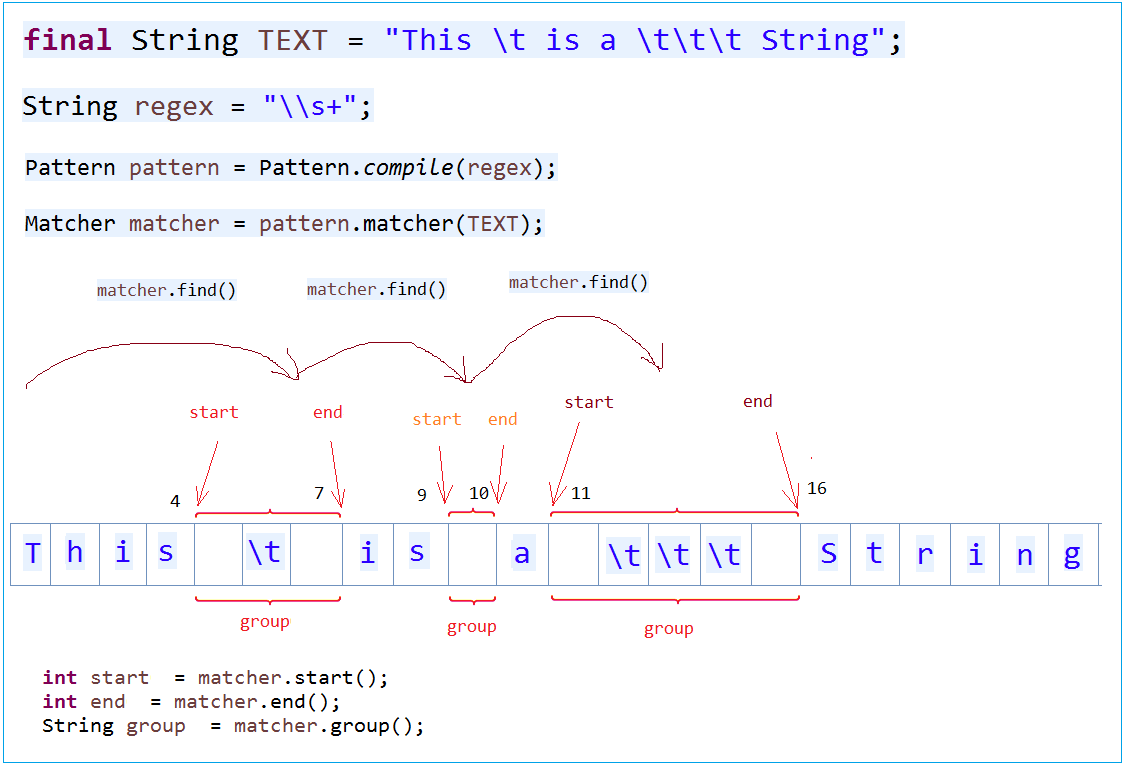MatcherFind.java
``````
package org.o7planning.tutorial.regex;

import java.util.regex.Matcher;
import java.util.regex.Pattern;

public class MatcherFind {

public static void main(String[] args) {

final String TEXT = "This \t is a \t\t\t String";

// Spaces appears one or more time.
String regex = "\\s+";

Pattern pattern = Pattern.compile(regex);

Matcher matcher = pattern.matcher(TEXT);

int i = 0;
while (matcher.find()) {
System.out.print("start" + i + " = " + matcher.start());
System.out.print(" end" + i + " = " + matcher.end());
System.out.println(" group" + i + " = " + matcher.group());
i++;
}

}
}
``````
Result of running example:
``````
start = 4 end = 7 group =
start = 9 end = 10 group =
start = 11 end = 16 group =
``````
Method Matcher.lookingAt()
MatcherLookingAt.java
``````
package org.o7planning.tutorial.regex;

import java.util.regex.Matcher;
import java.util.regex.Pattern;

public class MatcherLookingAt {

public static void main(String[] args) {
String country1 = "iran";
String country2 = "Iraq";

// Start by I followed by any character.
// Following is the letter a or e.
String regex = "^I.[ae]";

Pattern pattern = Pattern.compile(regex, Pattern.CASE_INSENSITIVE);

Matcher matcher = pattern.matcher(country1);

// lookingAt () searches that match the first part.
System.out.println("lookingAt = " + matcher.lookingAt());

// matches() must be matching the entire
System.out.println("matches = " + matcher.matches());

// Reset matcher with new text: country2
matcher.reset(country2);

System.out.println("lookingAt = " + matcher.lookingAt());
System.out.println("matches = " + matcher.matches());
}
}
``````

## 6- Group

A regular expression you can split into groups:
``````
// A regular expression
String regex = "\\s+=\\d+";

// Writing as three group, by marking ()
String regex2 = "(\\s+)(=)(\\d+)";

// Two group
String regex3 = "(\\s+)(=\\d+)";
``````
The group can be nested, and so need a rule indexing the group. The entire pattern is defined as the group 0. The remaining group described similar illustration below: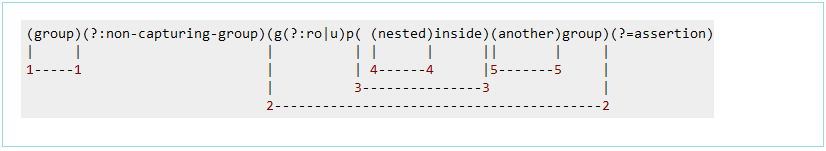Note: Use (?:Pattern) to inform Java does not see this as a group (None-capturing group)

From Java 7, you can define a named capturing group (?<name>pattern), and you can access the content matched with Matcher.group(String name). The regex is longer, but the code is more meaningful, since it indicates what you are trying to match or extract with the regex.

Named capturing group can also be access via Matcher.group(int group) with the same numbering scheme.

Internally, Java's implementation just maps from the name to the group number. Therefore, you cannot use the same name for 2 different capturing groups.
-
Let's look at an example using the named for the group (Java> = 7)
NamedGroup.java
``````
package org.o7planning.tutorial.regex;

import java.util.regex.Matcher;
import java.util.regex.Pattern;

public class NamedGroup {

public static void main(String[] args) {

final String TEXT = " int a = 100;float b= 130;float c= 110 ; ";

// Use (?<groupName>pattern) to define a group named: groupName
// Defined group named declare: using (?<declare>...)
// And a group named value: use: (?<value>..)
String regex = "(?<declare>\\s*(int|float)\\s+[a-z]\\s*)=(?<value>\\s*\\d+\\s*);";

Pattern pattern = Pattern.compile(regex);

Matcher matcher = pattern.matcher(TEXT);

while (matcher.find()) {
String group = matcher.group();
System.out.println(group);
System.out.println("declare: " + matcher.group("declare"));
System.out.println("value: " + matcher.group("value"));
System.out.println("------------------------------");
}
}
}
``````
Results of running the example:
``````
int a = 100;
declare:  int a
value:  100
------------------------------
float b= 130;
declare: float b
value:  130
------------------------------
float c= 110 ;
declare: float c
value:  110
------------------------------
``````
Just to clarify you can see the illustration below: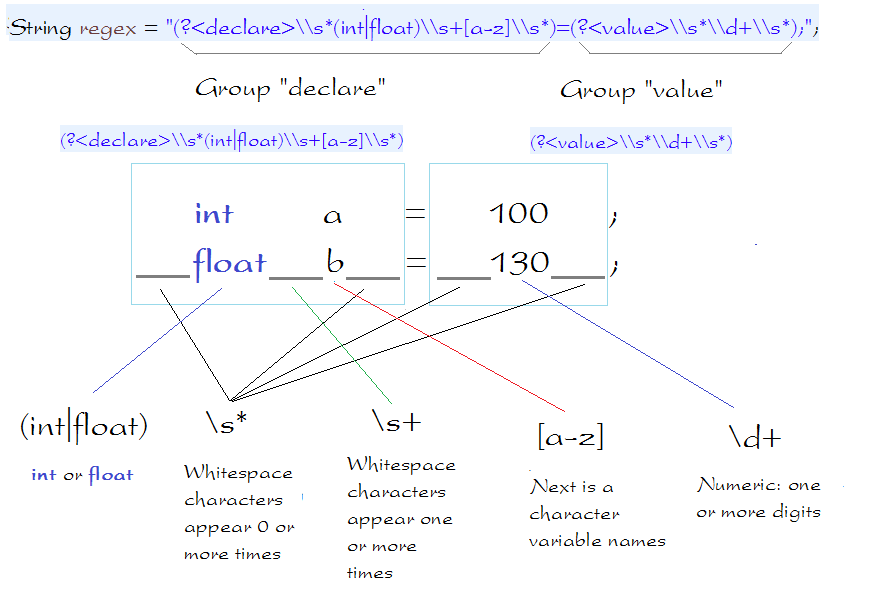## 7- Using Pattern, Matcher, Group and *?

In some situations *? very important, take a look at the following example:
``````
// This is a regex
// any characters appear 0 or more times,
// followed by ' and >
String regex = ".*'>";

// TEXT1 match the regex.
String TEXT1 = "FILE1'>";

// And TEXT2 match the regex
String TEXT2 = "FILE1'> <a href='http://HOST/file/FILE2'>";
``````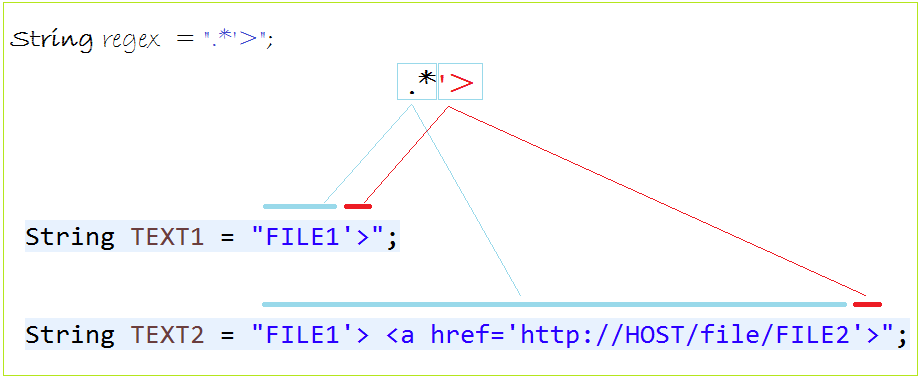*? will find the smallest match. We consider the following example:
NamedGroup2.java
``````
package org.o7planning.tutorial.regex;

import java.util.regex.Matcher;
import java.util.regex.Pattern;

public class NamedGroup2 {

public static void main(String[] args) {
String TEXT = "<a href='http://HOST/file/FILE1'>File 1</a>"
+ "<a href='http://HOST/file/FILE2'>File 2</a>";

// Java >= 7.
// Define group named fileName.
// *? ==> ? after a quantifier makes it a reluctant quantifier.
// It tries to find the smallest match.
String regex = "/file/(?<fileName>.*?)'>";

Pattern pattern = Pattern.compile(regex);
Matcher matcher = pattern.matcher(TEXT);

while (matcher.find()) {
System.out.println("File Name = " + matcher.group("fileName"));
}
}

}
``````
Results of running the example:
``````
File Name = FILE1
File Name = FILE2
``````

#### View more Tutorials:

Maybe you are interested

These are online courses outside the o7planning website that we introduced, which may include free or discounted courses.